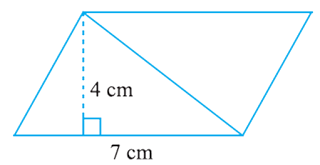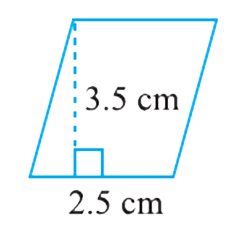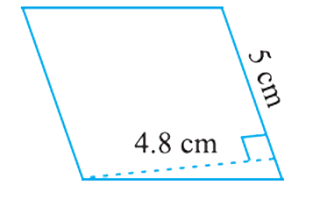# vs.eyeandcontacts.com

## Chapter 11 Perimeter and Area Exercise 11.2

Question 1: Find the area of each of the following parallelograms:
(a)Area of Parallelogram = height x base
= 4 x 7
= 28 cm²
(b)Area of Parallelogram = height x base
= 3 x 5
= 15 cm²
(c)Area of Parallelogram = height x base
= 3.5 x 2.5
= 8.75 cm²
(d)Area of Parallelogram = height x base
= 4.8 x 5
= 24 cm²
(e)

Area of Parallelogram = height x base
= 4.4 x 2
= 8.8 cm²
Question 2: Find the area of each of the following triangles:
(a)Area of triangle = 1/2 x base x height
= 1/2 x 4 x 3
= 12/2
= 6 cm²
(b)Area of triangle = 1/2 x base x height
= 1/2 x 5 x 3.2
= 16/2
= 8 cm²
(c)Area of triangle = 1/2 x base x height
= 1/2 x 3 x 4
= 12/2
= 6 cm²
(d)Area of triangle = 1/2 x base x height
= 1/2 x 3 x 2
= 6/2
= 3 cm²

Question 3: Find the missing values:

 S.No. Base Height Area of the Parallelogram a. 20 cm 246 cm2 b. 15 cm 154.5 cm2 c. 8.4 cm 48.72 cm2 d. 15.6 cm 16.38 cm2

(a) Given
Area of Parallelogram = 246 cm²
Base = 20 cm
Height = ?
= Height = Area/Base
= 246/20
= 12.3 cm
Therefore, height of the parallelogram is 12.3 cm.

(b)
Given
Area of Parallelogram = 154.5 cm²
Base = ?
Height = 15 cm
= Base = Area/Height
= 154.5/15
= 10.3 cm
Therefore, base of the parallelogram is 10.3 cm.

(c) Given
Area of Parallelogram = 48.72 cm²
Base = ?
Height = 8.4 cm
= Base = Area/Height
= 48.72/8.4
= 5.8 cm
Therefore, base of the parallelogram is 5.8 cm.

(d) Given
Area of Parallelogram = 16.38 cm²
Base = 15.6 cm
Height = ?
= Height = Area/Base
= 16.38/15.6
= 1.05 cm
Therefore, height of the parallelogram is 1.05 cm.

Question 4: Find the missing values:

 Base Height Area of Triangle 15 cm 87 cm2 31.4 mm 1256 mm2 22 cm 170.5 cm2

(a) Given
Area of triangle = 87 cm²
Base = 15 cm
Height = ?
= Height = 2 x Area/Base
= 2 x 87/15
= 174/15
= 11.6 cm
Therefore, the height of triangle is 11.6 cm.

(b) Given
Area of triangle = 1256 mm²
Base = ?
Height = 31.4 mm
= Base = 2 x Area/Height
= 2 x 1256/31.4
= 2512/31.4
= 80 mm
Therefore, the base of triangle is 80 mm.

Question 5: PQRS is a parallelogram (Fig 11.23). QM is the height from Q to SR and QN is the height from Q to PS. If SR = 12 cm and QM = 7.6 cm. Find:

(a) The area of the parallelogram PQRS
(b) QN, if PS = 8 cm
(a)
Area of Parallelogram = height x base
= SR x QM
= 12 x 7.6
= 91.2 cm²
Therefore, the area of parallelogram PQRS is 91.2 cm².

(b)

QN = ? If PS = 8 cm
Area = 91.2 cm²
Base = 8 cm
Height = ?
= Height = Area/Base
= 91.2/8
= Height = 11.4 cm
Therefore, QN is 11.4 cm.

Question 6: DL and BM are the heights on sides AB and AD respectively of parallelogram ABCD (Fig 11.24). If the area of the parallelogram is 1470 cm2, AB = 35 cm and AD = 49 cm, find the length of BM and DL.

Area = 1470 cm²
AB = 35 cm (base)
BM = ? (height)
DL = ? (height)
(i)
Area = 1470 cm²
Base = AB = 35 cm
Height = DL = ?
= Height = Area/Base
= 1470/35
= 42 cm
Therefore, DL is 42 cm.
(ii)
Area = 1470 cm²
Base = AD = 49 cm
Height = BM = ?
= Height = Area/Base
= 1470/49
= 30 cm
Therefore, BM is 30 cm.

Question 7: ΔABC is right angled at A (Fig 11.25). AD is perpendicular to BC. If AB = 5 cm, BC = 13 cm and AC = 12 cm, Find the area of ΔABC. Also find the length of AD.

AB = 5 cm
BC = 13 cm
AC = 12 cm
Area of   ⃤   ABC = 1/2 x base x height
= 1/2 x AB x AC
= 1/2 x 5 x 12
= 60/2
= 30 cm²
Therefore, the area of    ⃤   ABC is 30 cm².
Area = 30 cm²
Base = BC = 13 cm
Height = 2 x Area/Base
= 2 x 30/13
= 60/13
= 4 8/13
Therefore, AD is 4 8/13 cm.

Question 8: ΔABC is isosceles with AB = AC = 7.5 cm and BC = 9 cm (Fig 11.26). The height AD from A to BC, is 6 cm. Find the area of ΔABC. What will be the height from C to AB i.e., CE?

AB = AC = 7.5 cm
BC = 9 cm
Area of   ⃤   ABC= 1/2 x base x height
= 1/2 x BC x AD
= 1/2 x 9 x 6
= 54/2
= 27 cm²
Therefore, the area of   ⃤   ABC is 27 cm².
Area = 27 cm²
Base = 7.5 cm = AB
Height = ? = CE
Height = 2 x Area/Base
= 2 x 27/7.5
= 54/7.5
= 54/7.5 x 10/10
= 540/75
= 7.2 cm
Therefore, CE is 7.2 cm.